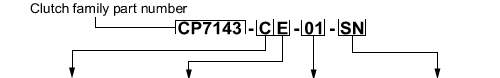## Part Numbering System

 New Part Number ReferencesDiaphragmSpring type Ratio Material Flywheel Type N = GRN(Green) L = LoR(Low Ratio) 01 = Aluminium CoverSteel Pressure PlateCarbon Type = Standard FN =Standard Flat O = ORA(Orange) H = HiR(High Ratio) 02 = Aluminium CoverSteel Pressure PlateCarbon Type = Heavy SN =Standard Stepped G = GRY(Grey) V = VHR(Very High Ratio) 03 = Steel CoverSteel Pressure PlateCarbon Type = Heavy FC =Flat with CFS C = CRV(Double Grey) E = EHR(Extra High Ratio) 05 = Titanium CoverSteel Pressure PlateCarbon Type = Heavy SC =Stepped with CFS S = SLV(Triple Orange) S = SHR(Super High Ratio) 06 = Titanium CoverTitanium Pressure PlateCarbon Type = Heavy SP =Stepped with CPS T  = TGY(Triple Grey) M = MHR(Mega High Ratio) FP =Flat with CPS D = GLD(Triple CRV) U = UHR(Ultra High Ratio)